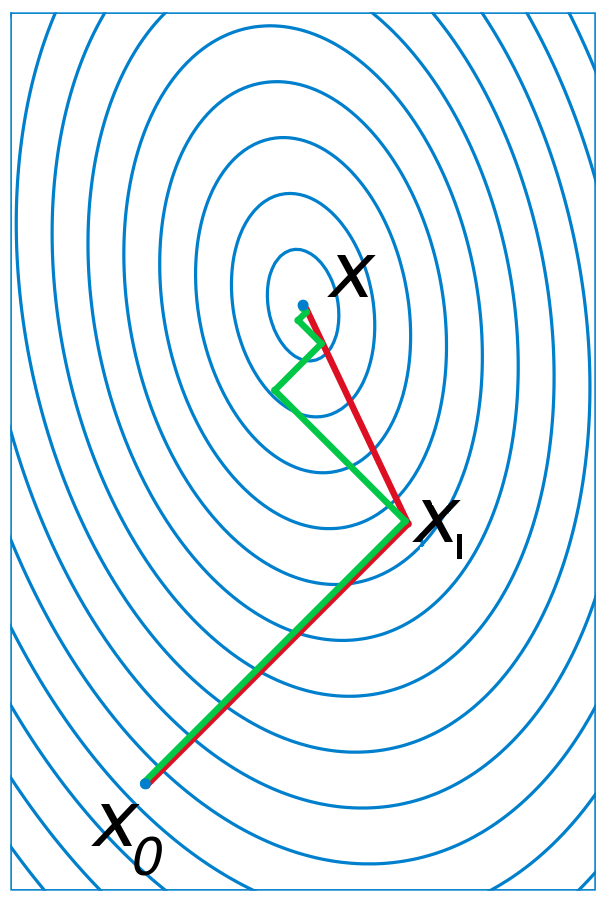# Quosi Newton Methods

## Newton’s Method

$$f(X) \approx f(X_k) + \nabla f(X_k) \cdot (X - X_k) + 0.5 \cdot (X - X_k) ^T \cdot \nabla ^2 f(X_k) \cdot (X - X_k)\tag{1}\label{eq1}$$.

$$\nabla f = g = \begin{pmatrix} \frac{\partial f}{\partial x_1} \\ \frac{\partial f}{\partial x_2} \\ \vdots \\ \frac{\partial f}{\partial x_N} \\ \end{pmatrix} \\$$

$$\nabla ^2 f = H = \begin{pmatrix} \frac{\partial ^2 f}{\partial x_1 ^2} & \frac{\partial ^2 f}{\partial x_1\partial x_2} & \cdots & \frac{\partial ^2 f}{\partial x_1\partial x_N}\\ \frac{\partial ^2 f}{\partial x_2\partial x_1} & \frac{\partial ^2 f}{\partial x_2 ^2} & \cdots & \frac{\partial ^2 f}{\partial x_2\partial x_N}\\ \vdots & \vdots & \ddots & \vdots\\ \frac{\partial ^2 f}{\partial x_N\partial x_1} & \frac{\partial ^2 f}{\partial x_N\partial x_2} & \cdots & \frac{\partial ^2 f}{\partial x_N ^2}\\ \end{pmatrix}$$

$\eqref{eq1}$ 的约等号两边分别对 X 求梯度，得到 $\nabla f(X) = g_k + H_k \cdot (X - X_k)$, 我们希望它的值为0，解出对应的 X，作为极值点的下一个估计 $X_{k+1} = X_k - H_k ^{-1} \cdot g_k$.

### Wolfe Conditions

1. $f(\mathbf{X}_k+\lambda\mathbf{p}_k)\leq f(\mathbf{X}_k)+c_1\lambda\mathbf{p}_k ^{\mathrm T}\nabla f(\mathbf{X}_k)$
2. $\mathbf{p}_k ^{\mathrm T}\nabla f(\mathbf{X}_k+\lambda\mathbf{p}_k) \geq c_2\mathbf{p}_k ^{\mathrm T}\nabla f(\mathbf{X}_k)$

1. 迭代之后的函数值确实减小了（此处考虑极小值问题，所以牛顿方向需要满足 $p_k ^T g_k < 0$），且至少以线性速率递减，c1 通常取一个很小的数，如 0.0001
2. 每次迭代之后，梯度以近似指数的速率缩小（考虑到两边均小于0），因而能向0趋近，c2 为衰减速率，因而取一个近似1的较大值即可，如 0.9

## Broyden–Fletcher–Goldfarb–Shanno algorithm

1. 给定初值 $X_0$ 和精度阈值e，k=0
2. 计算 $g_k, H_k$
3. 若 $||g_k|| < e$ 停止，否则计算牛顿方向 $\mathbf{p}_k = - H_k ^{-1} \cdot g_k$
4. 利用牛顿方向计算最优步长因子，$argmin_{\lambda} f(X_k + \lambda \mathbf{p}_k)$
5. k++, goto step 2

1. $s_k = X_{k+1} - X_k$
2. $y_k = g_{k+1} - g_k$
3. $B_k \approx H_k$

• 如果高阶导数均连续，则 H 矩阵为对称矩阵，参见 Clairaut’s theorem
• 保留正定性
• 碰巧有满足拟牛顿条件的特殊解： $\Delta B_k = \frac{y_k y_k ^T}{y_k ^T s_k} - \frac{B_k s_k s_k ^T B_k}{s_k ^T B_k s_k}$

$$B_{k+1} ^{-1} = \left (I-\frac { s_k y_k ^T} {y_k ^T s_k} \right ) B_{k} ^{-1} \left (I-\frac { y_k s_k ^T} {y_k ^T s_k} \right )+\frac {s_k s_k ^T} {y_k ^T \, s_k} \\ = B_k ^{-1} + \frac{(\mathbf{s}_k ^{\mathrm{T}}\mathbf{y}_k+\mathbf{y}_k ^{\mathrm{T}} B_k ^{-1} \mathbf{y}_k)(\mathbf{s}_k \mathbf{s}_k ^{\mathrm{T}})}{(\mathbf{s}_k ^{\mathrm{T}} \mathbf{y}_k) ^2} - \frac{B_k ^{-1} \mathbf{y}_k \mathbf{s}_k ^{\mathrm{T}} + \mathbf{s}_k \mathbf{y}_k ^{\mathrm{T}}B_k ^{-1}}{\mathbf{s}_k ^{\mathrm{T}} \mathbf{y}_k}$$

### L-BFGS

1. $\rho_k = \frac{1}{y_k ^Ts_k}$
2. $V_k = I - \rho_ky_ks_k ^T$
3. $D_k = B_k ^{-1}$

$$D\_{k+1} = (V\_k ^TV\_{k-1} ^T \cdots V\_{k-m+1} ^T)D\_{k-m+1}(V\_{k-m+1} \cdots V\_{k-1}V\_k) \\\\ + (V\_k ^TV\_{k-1} ^T \cdots V\_{k-m+2} ^T)(\rho\_{k-m+1}s\_{k-m+1}s\_{k-m+1} ^T)(V\_{k-m+2} \cdots V\_{k-1}V\_k) \\\\ + (V\_k ^TV\_{k-1} ^T \cdots V\_{k-m+3} ^T)(\rho\_{k-m+2}s\_{k-m+2}s\_{k-m+2} ^T)(V\_{k-m+3} \cdots V\_{k-1}V\_k) \\\\ + ... \\\\ + (V\_k ^TV\_{k-1} ^T)(\rho\_{k-2}s\_{k-2}s\_{k-2} ^T)(V\_{k-1}V\_k) \\\\ + V\_k ^T(\rho\_{k-1}s\_{k-1}s\_{k-1} ^T)V\_k \\\\ + \rho\_ks\_ks\_k ^T$$

## Conjugate Gradient Method

### Steepest Gradient Descent

1. 寻找函数在点 X 的梯度 $\nabla f(X)$
2. 沿梯度反方向作 line search，寻找更新步长 $argmin_{\lambda} f(X - \lambda \nabla f(X))$
3. 根据2的结果更新 X，重复1-2直至收敛### Approximate Linear Equation

$$f(X) = \frac12 X ^\mathrm{T} \mathbf{A}X - X ^\mathrm{T} \mathbf{b} , \quad X\in\mathbf{R} ^n.$$

$$\mathbf{AX_*} = \mathbf{b}\tag{2}\label{eq2}$$.

### A-conjugate Vectors

1. 如何选择共轭方向？
2. 如何计算每一步的更新步长？

### Iterative Method

1. 方向选择
1. 初始时，我们选取的更新方向为 $\mathbf{p}_0 = -\nabla{f(X_0)} = \mathbf{b} - \mathbf{A} X_0$，
2. 之后将依次选择接近于 $\mathbf{r}_k = \nabla{f(X_k)}$, 且与之前的更新方向均共轭的方向 $\mathbf{p}_k$
3. 更新的方法类似于正交基的选取，$\mathbf{p}_{k} = \mathbf{r}_{k} - \sum_{i < k}\frac{\mathbf{p}_i ^\mathrm{T} \mathbf{A} \mathbf{r}_{k}}{\mathbf{p}_i ^\mathrm{T}\mathbf{A} \mathbf{p}_i} \mathbf{p}_i$, 即需要从负梯度方向中减去它在之前各个更新方向中的 A-projection. 参见 Gram–Schmidt process.
2. 更新步长
1. 由于初始点选为 0 点，所以有 $X_k = \sum\limits_{i < k} \lambda_k \mathbf{p}_k$, 于是 $\mathbf{p}_k ^{\mathrm{T}} \mathbf{A} X_k = 0$. 即每一步的更新方向与此刻 X 所在的方向共轭。
2. 计算 $argmin_{\lambda_k} - \frac12 (X_k + \lambda_k \mathbf{p}_k) ^{\mathrm{T}} A (X_k + \lambda_k \mathbf{p}_k) + \mathbf{b}(X_k + \lambda_k \mathbf{p}_k)$
3. 令上式对 $\lambda_k$ 的偏导为0，并结合1中得出的共轭结论，容易得到更新步长的解析解：

$$\lambda_{k} = \frac{\mathbf{p}_k ^\mathrm{T} \mathbf{b}}{\mathbf{p}_k ^\mathrm{T} \mathbf{A} \mathbf{p}_k} = \frac{\mathbf{p}_k ^\mathrm{T} (\mathbf{r}_{k-1}+\mathbf{Ax}_{k-1})}{\mathbf{p}_{k} ^\mathrm{T} \mathbf{A} \mathbf{p}_{k}} = \frac{\mathbf{p}_{k} ^\mathrm{T} \mathbf{r}_{k-1}}{\mathbf{p}_{k} ^\mathrm{T} \mathbf{A} \mathbf{p}_{k}}$$

### Tuning

1. 计算负梯度方向
2. 根据 Fletcher-Reever 公式（或其它变种）计算方向变化量
3. 根据1,2的结果以及“上一个共轭方向”计算新的共轭方向
4. 在新的方向上作 line search 找到合适步长
5. 更新 X
6. 重复 1-5 直至收敛